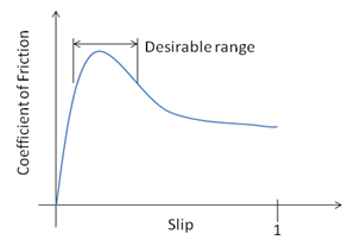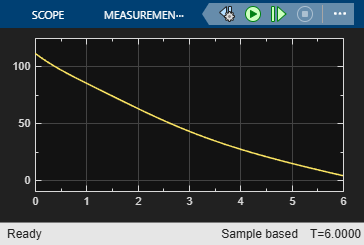# Anti-Lock Braking Using Extremum Seeking Control

This example shows how to use the extremum seeking control (ESC) to optimize braking torque for an anti-lock braking system (ABS).

### Anti-Lock Braking System

An anti-lock braking system prevents vehicle brakes from locking up by adjusting the braking torque for each wheel. For such a system, the following function defines the slip coefficient of a wheel.

`$slip=1-\frac{{\omega }_{w}}{{\omega }_{v}}$`

Here, ${\omega }_{w}$ is the wheel angular velocity and ${\omega }_{v}$ is the wheel angular velocity under a nonbraking condition (vehicle speed divided by wheel radius). Based on this equation, slip is zero when the wheel speed and vehicle speed are equal, and slip equals one when the wheel is locked (${\omega }_{w}$ is zero). A desirable slip value for braking is 0.2, which means that the number of wheel revolutions equals 0.8 times the number of revolutions under nonbraking conditions with the same vehicle velocity. This slip value maximizes the adhesion between the tire and road and minimizes the stopping distance for the available friction.

The friction coefficient $\mu$ between the tire and the road surface is a function of slip, known as the mu-slip curve.The frictional force ${F}_{f}$ acting on the circumference of the tire is the product of the friction coefficient $\mu$ multiplied by the weight on the wheel $\mathit{W}$. ${F}_{f}$ divided by the vehicle mass is equal to the vehicle deceleration, which you can integrate to obtain vehicle velocity.

Ideally, an anti-lock braking controller uses bang-bang control based upon the error between the actual slip and desired slip. The desired slip value is a constant and corresponds to the slip value for which the mu-slip curve reaches its peak value. For more information, see Modeling an Anti-Lock Braking System.

### Specify Vehicle Model Parameters

Define the following vehicle parameters for this example.

• `m` — Vehicle mass

• `W` — Vehicle weight

• `B` — Wheel damping torque coefficient

• `Rr` — Wheel radius

• `I` — Wheel inertia

```m = 400; W = m*9.81; B = 0.01; Rr = 0.3; I = 1;```

Also, specify the initial vehicle forward velocity `v0` and initial wheel angular velocity `x0`.

```v0 = 120/3.6; w0 = 400/3.6;```

### Extremum Seeking Control for Anti-Lock Braking System

For this ABS example, you design an extremum seeking controller that maximizes the friction coefficient, which is a function of the slip coefficient as shown in the following equation.

`$\mu =\frac{2{\mu }^{*}{\lambda }^{*}\lambda }{\left({\lambda }^{*2}+{\lambda }^{2}\right)}$`

Here, ${\mu }^{*}$ and ${\lambda }^{*}$ are the ideal friction and slip coefficients, respectively. The actual friction $\mu$ equals ${\mu }^{*}$ when the achieved slip coefficient $\lambda$ equals the ideal slip coefficient ${\lambda }^{*}$. The ABS achieves this objective of maximum deceleration, and hence the shortest stopping distance, by controlling the braking torque, which is a function of the slip and friction coefficients.

Simulink Control Design software implements the ESC algorithm using the Extremum Seeking Control block. For this example, open the `ExtremumSeekingControlABS` model, which includes this block along with an ABS system model.

```mdl = 'ExtremumSeekingControlABS'; open_system(mdl)```The output of the Extremum Seeking Control block is the slip coefficient $\lambda$. Since the ESC controller maximizes the value of $\mu$, use this value as the objective function input for the block.

Specify an initial guess for the slip coefficient.

`IC = 0.15;`

Also, specify the ideal slip coefficient `lambda_star` and ideal friction coefficient `mu_star`.

```lambda_star = 0.25; mu_star = 0.6;```

The Extremum Seeking Control block perturbs the parameter value using a modulation signal. It then demodulates the resulting change in the objective function signal before computing the parameter update. Configure the extremum seeking control parameters for this block.

First specify the number of parameters to be tuned (`N`) and the learning rate (`lr`).

```N = 1; lr = 0.3;```

Configure the demodulation and modulation signals by specifying their frequency (`omega`), phases (`phi_1` and `phi_2`), and amplitudes (`a` and `b`).

```omega = 0.7; % Forcing frequency a = 1; % Demodulation amplitude b = 0.02; % Modulation amplitude phi_1 = pi/2; % Demodulation phase phi_2 = 0; % Modulation phase```

For this example, use a low-pass filter to remove high-frequency noise from the demodulated signal and a high-pass filter to remove bias from the perturbed objective function signal. Specify the cutoff frequencies for these filters.

```omega_lpf = 1; omega_hpf = 0.5;```

### Simulate Anti-Lock Braking System

Simulate the model.

`sim(mdl);`

View the friction coefficient simulation result. Within two seconds, $\mu$ reaches its maximum value.

`open_system([mdl '/mu'])`View the vehicle velocity and the wheel angular velocity, both of which decrease to zero during the braking simulation.

`open_system([mdl '/velocity'])``open_system([mdl '/wheel velocity'])``bdclose('ExtremumSeekingControlABS')`

### Reference

 Ariyur, Kartik B., and Miroslav Krstić. Real Time Optimization by Extremum Seeking Control. Hoboken, NJ: Wiley Interscience, 2003.

## Related Topics